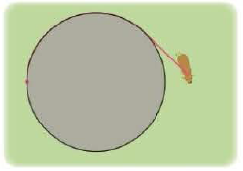Chapter 10.2, Problem 74E

Chapter
Section
Textbook Problem

# A cow is tied to a silo with radius r by a rope just long enough to reach the opposite side of the silo. Find the grazing area available for the cow.To determine

To find: The grazing area available for the cow tied to a silo with radius r .

Explanation

Given:

When a cow is tied to a silo with radius r . The rope is long enough to reach the opposite side of the silo.

The value of θ varies from 0 to π .

Calculation:

A portion of involute is traced when cow walks with the rope taut as shown in below figure 1.

When θ=π the arriving point of cow is (r,πr) .

The cow walks in semicircle of radius πr when fully extended with the rope. The cow traces another portion of the involute, it is the reflection about the x axis of the initial involute path.

The value of θ ranges from π to 0 .

From Figure 1, the total grazing area is 2(A1+A3) .

Here, A3 is the area of a circle.

The area A3 is one quarter of the area of a circle of radius πr .

Calculate the area of the quarter circle A3 .

A3=14π(πr)2=14π(π2r2)=14π3r2

The total area of the involute (A1+A2) is calculated by applying integral over the limits of the involute.

The right most point of the involute is (πr2,r) and the left most point of the involute is (r,πr) .

Calculate the total area of the involute (A1+A2) .

A1+A2=θ=ππ2ydxθ=0π2ydx=θ=ππ2ydx+π2θ=0ydx=θ=π0ydx . (1)

Therefore,

ydx=r(sinθθcosθθ2cos2θ)dθ=r2(θsinθcosθθ2cos2θ)dθ

Integrate the above equation on both sides

### Still sussing out bartleby?

Check out a sample textbook solution.

See a sample solution

#### The Solution to Your Study Problems

Bartleby provides explanations to thousands of textbook problems written by our experts, many with advanced degrees!

Get Started

#### Find the general indefinite integral. (1+rr)2dr

Single Variable Calculus: Early Transcendentals, Volume I

#### (x3y2z2)2

Applied Calculus for the Managerial, Life, and Social Sciences: A Brief Approach

#### In Problems 7-10, solve the systems of equations by substitution.

Mathematical Applications for the Management, Life, and Social Sciences

#### Differentiate the function. f(v)=v32vevv

Single Variable Calculus: Early Transcendentals

#### sinx=ex+ex2

Study Guide for Stewart's Single Variable Calculus: Early Transcendentals, 8th